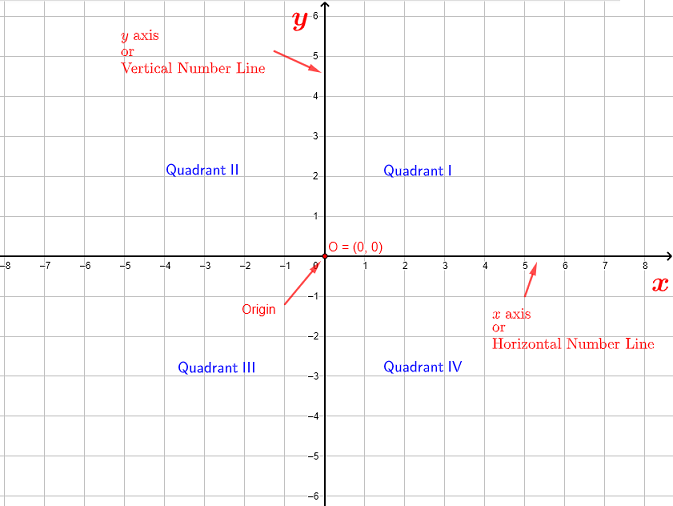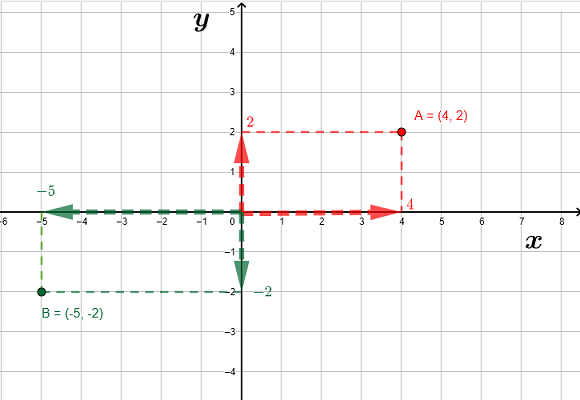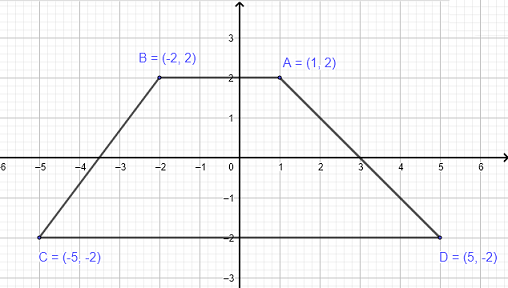# Rectangular Coordinate System in a Plane

The use of rectangular coordinate system is presented along with examples, questions and their solutions.

## Rectangular Coordinate System in a Plane

A rectangular coordinate system in a Plane is used to plot points having an $x$ coordinate and a $y$ coordinate. A vertical number line, also called the y-axis, and a horizontal number line, also called x-axis, intersecting at a right angle form a system of coordinates in a plane as shown in figure 1 below. The point of intersection of the x and y axes is called the origin of the system of coordinates.
The x and y axes split the plane into four quadrants as noted in the figure above.Fig.1 - Rectangular Coordinate System in a Plane Note that the rectangular coordinate system is also called the Cartesian coordinate system .

## Point Plotting in Rectangualar Coordinate System

Each point in the plane corresponds to an ordered pair $(x,y)$ , where $x$ and $y$ are real numbers. $x$ and $y$ are called the coordinates of the point where the $x$ coordinate represents the directed distance from the y axis to the point and the $y$ coordinate represent the directed distance from the x-axis to the point.
Example
In the example below, the $x$ coordinate of point $A$ is $4$ and therefore positive, hence point $A$ is located to the right of the y-axis in the direction of the arrow of the x-axis.
The $x$ coordinate of point $B$ is $-5$ and therefore negative, hence point $B$ is located to the left of the y-axis in the direction opposite to the arrow of the x-axis..
The $y$ coordinate of point $A$ is $2$ and therefore positive, hence point $A$ is located above the x-axis in the direction of the arrow of the y-axis..
The $y$ coordinate of point $B$ is $-2$ and therefore negative, hence point $B$ is located above the x-axis in the direction opposite to the arrow of the y-axis..Fig.2 - Example of Point Plotting in a Rectangular Coordinate System More practice on plotting points in rectangular coordinate system is included.

## Signs of Coordinates and Quadrants

The signs of the $x$ and $y$ coordinates of a given point, gives enough information to find the quadrant of that point without plotting it.
Example
Points $A = (4,2)$ and $C = (-4,3)$ are both located above the x axis because their $y$ coordinates $2$ and $3$ are both positive. But point $A$ is on the right of the y axis and hence in quadrant I because its $x$ coordinate $4$ is positive.
The $x$ coordinate of $C$, which is $-4$, is negative and therefore point $C$ is to the left of the y axis, hence located in quadrant II.
Similar remarks could be made about points $E = (-4,-2)$ in quadrant III and $G = (4,-2)$ in quadrant IV.
Conclusion
Given a point with coordinates $x$ and $y$, and without plotting the point, we can find the quadrant where the point will be located from the signs of $x$ and $y$.
If $x \gt 0$ and $y \gt 0$, the point is in quadrant I
If $x \lt 0$ and $y \gt 0$, the point is in quadrant II
If $x \lt 0$ and $y \lt 0$, the point is in quadrant III
If $x \gt 0$ and $y \lt 0$, the point is in quadrant IV

## Point on the x and y Axes

Any point whose $x$ coordinate is equal to zero, is located in the y axis because its distance from the y axis is equal to zero.
Example
Points $A = (0,3)$, $D = (0,-2)$ and $E = (0,-4)$ all have the $x$ coordinate equal to zero and are therefore located in the y axis. (see figure 4 below)

Any point whose $y$ coordinate is equal to zero, is located in the x axis because its distance from the x axis is equal to zero.
Example
Points $G = (6,0)$, $C = (-2,0)$ and $B = (-8,0)$ all have the $y$ coordinate equal to zero and are therefore located in the x axis. (see figure 4 below)

## Questions

### Part A

Plot the following points: $A = (0,0) \; , \; B = (-4,3) \; , \; C = (0,-4) \; ; \; D = (5,-5) \; ; \; E = (-3,0) \; ; \; F = (-2,-3) \; ; \; G = (4,0) \; ; \; H = (2,5)$

### Part B

Give the coordinates of all points plotted in the graph below.### Part C

Without plotting the points given below; in which quadrant or axis is each of the following points located?
$A = (-32,-89) \; , \; B = (0,45) \; , \; C = (-88,0) \; ; \; D = (57,89) \; ; \; E = (0,-77) \; ; \; F = (45,-38) \; ; \; G = (49,0) \; ; \; H = (-90,-56)$

### Part D

Graph each of the following group of points, link the points in the order that they are given and describe each quadrilateral obtained.
Group 1: $A = (2,2) \; , \; B = (-4,2) \; , \; C = (-4,-1) \; , \; D = (2,-1)$
Group 2: $A = (1,2) \; , \; B = (-2,2) \; , \; C = (-5,-2) \; , \; D = (5,-2)$
Group 3: $A = (0,4) \; , \; B = (-2,2) \; , \; C = (0,-4) \; , \; D = (2,2)$

## Solutions to the Above Questions

### Part A### Part B

$A = (0,1)$, $B = (2,0)$, $C = (1,3)$, $D = (-1,-1)$, $E = (1,-3)$, $F = (-3,1)$, $G = (0,-4)$, $H = (-3,0)$

### Part C

$A = (-32,-89)$ in quadrant III
$B = (0,45)$ on the y axis, above the x axis
$C = (-88,0)$ on the x axis, to the left the y axis
$D = (57,89)$ in quadrant I
$E = (0,-77)$ on the y axis, below the x axis
$F = (45,-38)$ in quadrant IV
$G = (49,0)$ on the x axis, to the right the y axis
$H = (-90,-56)$ in quadrant III

### Part D

Group 1: $A = (2,2) \; , \; B = (-4,2) \; , \; C = (-4,-1) \; , \; D = (2,-1)$
The four given points form a rectangle as shown below.Group 2: $A = (1,2) \; , \; B = (-2,2) \; , \; C = (-5,-2) \; , \; D = (5,-2)$
The four given points form a trapezoid as shown below.Group 3: $A = (0,4) \; , \; B = (-2,2) \; , \; C = (0,-4) \; , \; D = (2,2)$
The four given points form a kite as shown below.